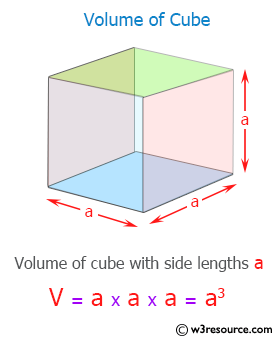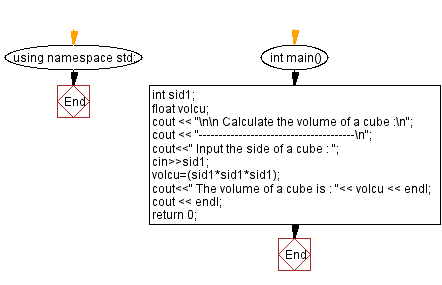﻿ C++ Exercises: Calculate the volume of a cube - w3resource

# C++ Exercises: Calculate the volume of a cube

## C++ Basic: Exercise-15 with Solution

Write a C++ program that calculates the volume of a cube.

Pictorial Presentation:Sample Solution:

C++ Code :

``````#include <iostream>
using namespace std;

int main()
{
int sid1;
float volcu;
cout << "\n\n Calculate the volume of a cube :\n";
cout << "---------------------------------------\n";
cout<<" Input the side of a cube : ";
cin>>sid1;
volcu=(sid1*sid1*sid1);
cout<<" The volume of a cube is : "<< volcu << endl;
cout << endl;
return 0;
}
``````

Sample Output:

``` Calculate the volume of a cube :
---------------------------------------
Input the side of a cube : 5
The volume of a cube is : 125
```

Flowchart:C++ Code Editor:

What is the difficulty level of this exercise?

﻿

## C++ Programming: Tips of the Day

Why is there no std::stou?

The most pat answer would be that the C library has no corresponding "strtou", and the C++11 string functions are all just thinly veiled wrappers around the C library functions: The std::sto* functions mirror strto*, and the std::to_string functions use sprintf.

Ref: https://bit.ly/3wtz2qA

We are closing our Disqus commenting system for some maintenanace issues. You may write to us at reach[at]yahoo[dot]com or visit us at Facebook